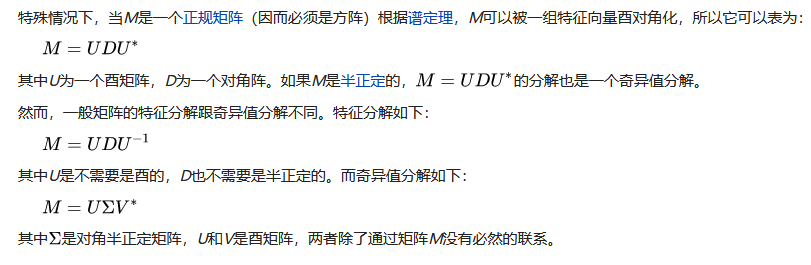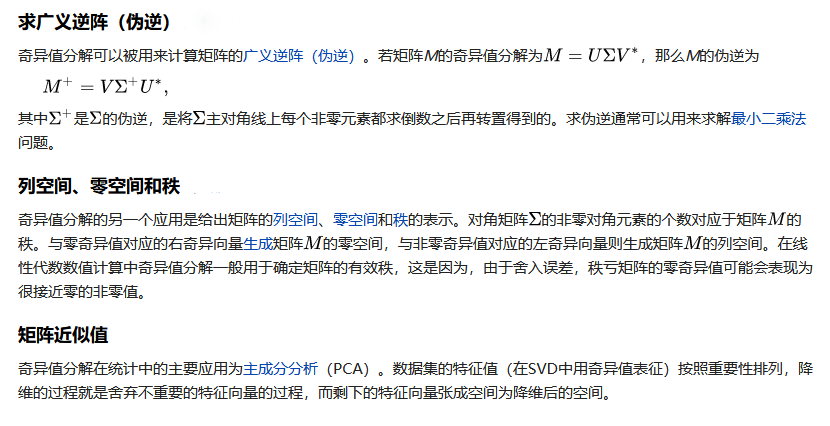• 矩阵对角化python
2020-12-03 11:18:38

我使用scipy中的linalg来得到155X156矩阵的e值和特征向量。然而，与矩阵相比，特征值的阶数似乎是随机的。我希望第一个特征值对应于矩阵中的第一个数。请看下面我的例行程序。我首先读取的是一个包含所有浮点数的文件(1u1o.dat)2533297.650278 -2373859.531153

37695.845843 425449.129032

然后我把它们当作数组来读

^{pr2}\$

然后重塑成156X156矩阵。我想把所有的特征值和相应的特征向量按矩阵读取的顺序打印出来。我知道最后我的特征值(156个数字)应该从小到高列出，而不是像现在的程序那样随机。当然，对应特征向量的阶数也是一样的。有人能帮我吗？在

谢谢。在from scipy import linalg

from scipy.linalg import *

file2 = open('1_1f.dat', 'w')

with open('1_1o.dat', 'rU') as file:

nums2 = np.array(File)

nums2 = [float(i.rstrip('\n')) for i in nums2.split()]

nums2 = np.reshape(nums2, (156, 156))

print eig(nums2)

print >> file2, eig(nums2)

file2.close()

更多相关内容
• 每个方阵都对应一个线性变换，矩阵对角化的本质是找线性变化的特征值和特征向量。线性变换可以代表一种操作（如坐标系的转动）或者代表一个力学量（如量子力学中的动量、角动量等），运用非常广泛。
• 线性代数矩阵对角化中一个定理的超长证明！------------------------------------------
• 矩阵对角化（Diagonalizing a Matrix）1.1 矩阵的优点1.2 求解一般矩阵的特征值和特征向量1.3 矩阵对角化1.4 计算 AkA^kAk1.5 矩阵对角化的注意事项 1.矩阵对角化（Diagonalizing a Matrix） 笔记参考来源：...

# 1.矩阵对角化（Diagonalizing a Matrix）

## 1.1 对角矩阵的优点对角矩阵优点1：

我们观察到对角矩阵只是对标准基进行了缩放，而没有进行旋转（这里的性质和特征向量有些类似！！！）这大大提高了线性变换的确定性（尤其对于高维空间中的线性变换），同时也大大简化了计算量对角矩阵优点2：

特别容易求出对角矩阵的逆矩阵## 1.2 求解一般矩阵的特征值和特征向量

我们将一般矩阵化为对角矩阵我们观察到一般矩阵相较于对角矩阵，后者的线性变换较为复杂我们求出上述矩阵的特征向量和特征值

求解矩阵的特征向量、特征值
方法一：
A = [ 5 / 4 3 / 4 3 / 4 5 / 4 ]   A x ⃗ = λ x ⃗   ( A − λ I ) x ⃗ = 0 ⃗   A − λ I = [ 5 / 4 − λ 3 / 4 3 / 4 5 / 4 − λ ]   d e t ( A − λ I ) = 0   d e t ( A − λ I ) = ( 5 4 − λ ) 2 − ( 3 4 ) 2 = 0   λ 1 = 1 2 、 λ 2 = 2   A x ⃗ 1 = 1 2 x ⃗ 1 、 A x ⃗ 2 = 2 x ⃗ 2   [ 5 / 4 3 / 4 3 / 4 5 / 4 ] [ a 1 a 2 ] = 1 2 [ a 1 a 2 ] 、 [ 5 / 4 3 / 4 3 / 4 5 / 4 ] [ a 3 a 4 ] = 2 [ a 3 a 4 ]   a 1 = − a 2 、 a 3 = a 4   W e   t a k e   a 1 = − 1 、 a 2 = 1 、 a 3 = 1 、 a 4 = 1   x ⃗ 1 = [ 1 1 ] 、 x ⃗ 2 = [ − 1 1 ] A=\begin{bmatrix}5/4 & 3/4\\ 3/4 & 5/4\end{bmatrix}\\ ~\\ A\vec{x}=\lambda \vec{x}\\ ~\\ (A-\lambda I)\vec{x}=\vec{0}\\ ~\\ A-\lambda I=\begin{bmatrix}5/4-\lambda & 3/4\\ 3/4 & 5/4-\lambda\end{bmatrix}\\ ~\\ det(A-\lambda I)=0\\ ~\\ det(A-\lambda I)=(\frac{5}{4}-\lambda)^2-(\frac{3}{4})^2=0\\ ~\\ \lambda_1=\frac{1}{2}、\lambda_2=2\\ ~\\ A\vec{x}_1=\frac{1}{2}\vec{x}_1、A\vec{x}_2=2\vec{x}_2\\ ~\\ \begin{bmatrix} 5/4 & 3/4\\ 3/4 & 5/4 \end{bmatrix} \begin{bmatrix} a_1\\ a_2 \end{bmatrix}=\frac{1}{2} \begin{bmatrix} a_1\\ a_2 \end{bmatrix}、 \begin{bmatrix} 5/4 & 3/4\\ 3/4 & 5/4 \end{bmatrix} \begin{bmatrix} a_3\\ a_4 \end{bmatrix}=2 \begin{bmatrix} a_3\\ a_4 \end{bmatrix}\\ ~\\ a_1=-a_2、a_3=a_4\\ ~\\ We\ take\ a_1=-1、a_2=1、a_3=1、a_4=1\\ ~\\ \vec{x}_1= \begin{bmatrix} 1\\ 1 \end{bmatrix}、 \vec{x}_2= \begin{bmatrix} -1\\ 1 \end{bmatrix}
方法二：
A = [ 5 / 4 3 / 4 3 / 4 5 / 4 ]   d e t   A = ( 5 4 ) 2 − ( 3 4 ) 2 = 1 = λ 1 λ 2   t r   A = 5 4 + 3 4 = 5 2 = λ 1 + λ 2   { λ 1 λ 2 = 1 λ 1 + λ 2 = 5 2   λ 1 = 1 2 、 λ 2 = 2   A x ⃗ 1 = 1 2 x ⃗ 1 、 A x ⃗ 2 = 2 x ⃗ 2   [ 5 / 4 3 / 4 3 / 4 5 / 4 ] [ a 1 a 2 ] = 1 2 [ a 1 a 2 ] 、 [ 5 / 4 3 / 4 3 / 4 5 / 4 ] [ a 3 a 4 ] = 2 [ a 3 a 4 ]   a 1 = − a 2 、 a 3 = a 4   W e   t a k e   a 1 = − 1 、 a 2 = 1 、 a 3 = 1 、 a 4 = 1   x ⃗ 1 = [ 1 1 ] 、 x ⃗ 2 = [ − 1 1 ] A=\begin{bmatrix}5/4 & 3/4\\ 3/4 & 5/4\end{bmatrix}\\ ~\\ det\ A=(\frac{5}{4})^2-(\frac{3}{4})^2=1=\lambda_1\lambda_2\\ ~\\ tr\ A=\frac{5}{4}+\frac{3}{4}=\frac{5}{2}=\lambda_1+\lambda_2\\ ~\\ \begin{cases} \lambda_1\lambda_2=1\\ \lambda_1+\lambda_2=\frac{5}{2} \end{cases}\\ ~\\ \lambda_1=\frac{1}{2}、\lambda_2=2\\ ~\\ A\vec{x}_1=\frac{1}{2}\vec{x}_1、A\vec{x}_2=2\vec{x}_2\\ ~\\ \begin{bmatrix} 5/4 & 3/4\\ 3/4 & 5/4 \end{bmatrix} \begin{bmatrix} a_1\\ a_2 \end{bmatrix}=\frac{1}{2} \begin{bmatrix} a_1\\ a_2 \end{bmatrix}、 \begin{bmatrix} 5/4 & 3/4\\ 3/4 & 5/4 \end{bmatrix} \begin{bmatrix} a_3\\ a_4 \end{bmatrix}=2 \begin{bmatrix} a_3\\ a_4 \end{bmatrix}\\ ~\\ a_1=-a_2、a_3=a_4\\ ~\\ We\ take\ a_1=-1、a_2=1、a_3=1、a_4=1\\ ~\\ \vec{x}_1= \begin{bmatrix} 1\\ 1 \end{bmatrix}、 \vec{x}_2= \begin{bmatrix} -1\\ 1 \end{bmatrix}## 1.3 矩阵对角化

特征向量矩阵 X X
X = [ x ⃗ 1 x ⃗ 2 ] = [ 1 − 1 1 1 ] X=[\vec{x}_1\quad\vec{x}_2]= \begin{bmatrix} 1 & -1\\ 1 & 1 \end{bmatrix}

特征值矩阵 Λ \Lambda
Λ = [ λ 1 0 0 λ 2 ] = [ 2 0 0 1 / 2 ] \Lambda= \begin{bmatrix} \lambda_1 & 0\\ 0 & \lambda_2 \end{bmatrix}= \begin{bmatrix} 2 & 0\\ 0 & 1/2 \end{bmatrix}
特征向量矩阵的逆矩阵 X − 1 X^{-1}X − 1 = 1 d e t   X [ 1 1 − 1 1 ] = 1 2 [ 1 1 − 1 1 ] = [ 1 / 2 1 / 2 − 1 / 2 1 / 2 ] X^{-1}=\frac{1}{det\ X} \begin{bmatrix} 1 & 1\\ -1 & 1 \end{bmatrix}=\frac{1}{2} \begin{bmatrix} 1 & 1\\ -1 & 1 \end{bmatrix}= \begin{bmatrix} 1/2 & 1/2\\ -1/2 & 1/2 \end{bmatrix}

将矩阵 A A 进行分解
A = X Λ X − 1 = [ 1 − 1 1 1 ] [ 2 0 0 1 / 2 ] [ 1 / 2 1 / 2 − 1 / 2 1 / 2 ] A=X\Lambda X^{-1}= \begin{bmatrix} 1 & -1\\ 1 & 1 \end{bmatrix} \begin{bmatrix} 2 & 0\\ 0 & 1/2 \end{bmatrix} \begin{bmatrix} 1/2 & 1/2\\ -1/2 & 1/2 \end{bmatrix}

其中 X X 是由矩阵的各个特征向量组成的矩阵、 Λ \Lambda 是由矩阵的各个特征值组成的对矩阵 X 、 Λ 、 X − 1 X、\Lambda、X^{-1} 对应的线性变换过程的可视化矩阵 X − 1 X^{-1} 将特征向量转换到了标准基的位置（我们将此时的特征向量称其为特征基矩阵 Λ \Lambda 特征基缩放了特征值大小矩阵 X X 将缩放后的特征基转换到特征向量的原本位置（原标准基的坐标系下）## 1.4 计算 A k A^k例子：## 1.5 矩阵对角化的注意事项可逆性与可对角化性无关！！！展开全文特征值 特征向量 特征基
•matlab
• 科学出版社线性代数自测题之第5章矩阵对角化自测题答案
• 为了提高高阶容积卡尔曼滤波器(CKF)的滤波性能, 提出一种基于矩阵对角化变换的高阶CKF 算法. 该算法基于高阶容积准则, 利用矩阵对角化变换代替标准高阶CKF 中的Cholesky 分解, 使得协方差矩阵分解后的平方根矩阵保留...
• ## 矩阵对角化方法

千次阅读 2020-12-30 20:10:59
1矩阵对角化方法摘要：本文给出了一种不同于传统方法的矩阵对角化方法，利用矩阵的初等变换，先求出矩阵的特征根与特征向量，接着再判断矩阵是否可对角化。关键词：矩阵特征根特征向量对角化...

1

矩阵对角化方法

摘要：

本文给出了一种不同于传统方法的矩阵对角化方法，利用矩阵的初等变换，先求出矩阵的特征根与特征向

量，接着再判断矩阵是否可对角化。

关键词：

矩阵

特征根

特征向量

对角化

The Methods of the Diagonalization of the Matrix

g

Abstract:

In this paper, the method of the diagonalization of the matrix is given, which is different from the traditional

methods. According to using the elementary transformation of the matrix, first of all, The author obtains the characteristic

roots and the characteristic vectors, then judge the diagonalization of the matrix.

Key words:

Matrix; Characteristic roots; Characteristic vectors; Diagonalization

1

、引言

对角化后的矩阵在计算和应用等方面比一般矩阵更具优越性，

而矩阵对角化方法

有很多，

如对于对称矩阵可以将其看成二次型所对应的矩阵，

通过配方法将其化为标

准形从而实现矩阵的对角化，再如通过求解特征根和特征向量方法，首先求解

0

|

|

A

E

得特征根

i

，然后对每一个

i

，解方程组

0

)

(

X

A

E

i

得特征向量，即

寻找一个可逆矩阵

T

，使得

AT

T

1

,

其中

为对角阵，于是可得

1

T

T

A

，从而

1

T

T

A

n

n

,

在这个对角化过程中，

中的元素即为矩阵

A

的特征根，

T

中每个列向

量即为矩阵

A

的属于每个特征根的特征向量。

本文主要介绍一种异于传统方法的矩阵

对角化方法，

即将矩阵的特征矩阵经过一系列初等变换将其化为上三角形矩阵或对角

形矩阵从而得到矩阵的特征根与特征向量，同时判断矩阵是否可对角化。

2

、讨论对于有

n

个特征单根的

n

阶方阵

1

.

2

基本原理

引理

1

：设

A

是秩为

r

n

m

阶矩阵，且

展开全文• - [矩阵对角化](#矩阵对角化) - [SVD分解](#svd分解) - [参考链接](#参考链接) 矩阵对角化 矩阵的相似 设 A\boldsymbol{A}A、 B\boldsymbol{B}B 为两个nnn阶矩阵，若存在可逆矩阵 P\boldsymbol{P}P，使得...

## 矩阵对角化

矩阵的相似

A \boldsymbol{A} B \boldsymbol{B} 为两个 n n 阶矩阵，若存在可逆矩阵 P \boldsymbol{P} ，使得
P − 1 A P = B \boldsymbol{P}^{^{-1}}\boldsymbol{A}\boldsymbol{P}=\boldsymbol{B}
则称 A \boldsymbol{A} B \boldsymbol{B} 相似。特别的，如果 B \boldsymbol{B} 为对角形矩阵，则称 A \boldsymbol{A} 可(相似)对角化

n n 阶矩阵 A A 可对角化的充要条件： A \boldsymbol{A} n n 个线性无关的特征向量 X 1 , X 2 , . . . X n \boldsymbol{X_{1}},\boldsymbol{X_{2}},...\boldsymbol{X_{n}} 。具体的证明我们不在此展开，只做几点说明：

1. n n 阶矩阵 A \boldsymbol{A} m m 个不同特征值（方程 ∣ λ E − A ∣ = 0 \left | \lambda \boldsymbol{E} -\boldsymbol{A}\right |=0 m m 个不同实根），则不同特征值对应的特征向量线性无关
2. 存在 A \boldsymbol{A} 属于某个特征值的线性无关特征向量
3. P − 1 A P = d i a g ( λ 1 ， λ 2 ， . . . λ n ) \boldsymbol{P}^{^{-1}}\boldsymbol{A}\boldsymbol{P}=diag(\lambda _{1}，\lambda _{2}，...\lambda _{n}) 时， d i a g ( λ 1 ， λ 2 ， . . . λ n ) diag(\lambda _{1}，\lambda _{2}，...\lambda _{n}) n n 个主对角元是 A \boldsymbol{A} n n 个特征值（含重根）； A \boldsymbol{A} 分别属于 λ 1 ， λ 2 ， . . . λ n \lambda _{1}，\lambda _{2}，...\lambda _{n} 的线性无关特征向量 X 1 , X 2 , . . . X n \boldsymbol{X_{1}},\boldsymbol{X_{2}},...\boldsymbol{X_{n}} 构成了可逆矩阵 P \boldsymbol{P} 的列向量

实对称矩阵的对角化

考虑实对称矩阵的对角化，有如下结论：

1. n n 阶实对称矩阵 A \boldsymbol{A} 的特征值都是实数（证明思路：对表达式 A X = λ X \mathbf{AX=\lambda X} 左乘复特征向量的共轭转置 X ˉ T \mathbf{\bar{X}^{T}} ，推出 λ \lambda 为实数）
2. 实对称矩阵 A \boldsymbol{A} 不同特征值对应的特征向量正交（证明思路：列出 A \boldsymbol{A} 两组不同的特征方程，分别左乘另一个复特征向量的共轭转置，两端取转置后方程相减）
3. 属于 A \boldsymbol{A} 的同一个特征值的一组线性无关特征向量不一定正交，但可用施密特正交方法将其正交化
4. 一定存在正交矩阵 Q \mathbf{Q} （满足 Q − 1 = Q T \mathbf{Q}^{-1}=\mathbf{Q}^{T} ）,使得 Q − 1 A Q \boldsymbol{Q}^{^{-1}}\boldsymbol{A}\boldsymbol{Q} 为对角形矩阵
5. n n 阶实对称矩阵 A \boldsymbol{A} 存在 n n 个正交的单位特征向量

## SVD分解

特征值分解只能用于可逆方阵，奇异值分解（SVD）适用于任意 m × n \mathit{m}\times\mathit{n} 矩阵，定义如下：

A \boldsymbol{A} 是一个 m × n \mathit{m}\times\mathit{n} 矩阵，则存在一个分解，使得
A = U Σ V ∗ \boldsymbol{A}=\boldsymbol{U}\Sigma\boldsymbol{V}^{*}
其中 U \boldsymbol{U} m × m m\times m 酉矩阵（满足 U − 1 = U ∗ \boldsymbol{U^{-1}}=\boldsymbol{U}^{*} ）, Σ \Sigma m × n m\times n 非负实对称矩阵， V ∗ \boldsymbol{V}^{*} V \boldsymbol{V} 的共轭转置，是 n × n n\times n 酉矩阵.

求解 U \boldsymbol{U} , Σ \Sigma , V \boldsymbol{V} 矩阵

给定一个 A m × n \boldsymbol{A_{m\times n}} 的奇异值分解，有：
A ∗ A = V Σ ∗ U ∗ U Σ V ∗ = V ( Σ ∗ Σ ) V ∗ \boldsymbol{A^{*}A}=\boldsymbol{V}\Sigma^{*}\boldsymbol{U}^{*}\boldsymbol{U}\Sigma\boldsymbol{V}^{*}=\boldsymbol{V}(\Sigma^{*}\Sigma)\boldsymbol{V}^{*}
A A ∗ = U Σ V ∗ V Σ ∗ U ∗ = U ( Σ Σ ∗ ) U ∗ \boldsymbol{AA^{*}}=\boldsymbol{U}\Sigma\boldsymbol{V}^{*}\boldsymbol{V}\Sigma^{*}\boldsymbol{U}^{*}=\boldsymbol{U}(\Sigma\Sigma^{*})\boldsymbol{U}^{*}
关系式右边描述了关系式左边的特征值分解，于是：

• A ∗ A \boldsymbol{A^{*}A} n n 个特征向量（右奇异向量）组成了 V \boldsymbol{V} 的列向量
• A A ∗ \boldsymbol{AA^{*}} m m 个特征向量（左奇异值向量）组成了 U \boldsymbol{U} 的列向量
• Σ \Sigma 的非零对角元（非零奇异值）是 A ∗ A \boldsymbol{A^{*}A} A A ∗ \boldsymbol{AA^{*}} 的非零特征值（为啥相同？）的平方根，即 Σ = [ σ 1 0 0 0 0 σ 2 ⋯ 0 0 0 ⋱ σ r ⋮ ⋮ ⋮ ⋮ ] m × n \Sigma=\begin{bmatrix} \sigma_{1}& 0& 0& 0 \\ 0& \sigma_{2}& \cdots& 0 \\ 0& 0& \ddots& \sigma_{r} \\ \vdots& \vdots& \vdots& \vdots \end{bmatrix}_{m\times n} ，其中 r = r a n k ( A ) r=rank(\boldsymbol{A}) （思考为什么？）且 m > r \mathit{m}>\mathit{r} σ i = λ i ( i = 1 , 2 ⋯ r ) \sigma _{i}=\sqrt{\lambda _{i}}(i=1,2\cdots r)

补充1： A A T \boldsymbol{AA^{T}} 性质

• 对称性： ( A T A ) T = A A T (\boldsymbol{A^{T}A})^{T}=\boldsymbol{AA^{T}}
• 半正定性：对任意非零向量 x n × 1 x_{n\times1} ，有 x T ( A T A ) x = ( A x ) T ( A x ) ⩾ 0 \boldsymbol{x^{T}}(\boldsymbol{A^{T}A})\boldsymbol{x}=(\boldsymbol{Ax})^{T}(\boldsymbol{Ax})\geqslant 0

补充2：奇异值分解 Vs.特征值分解补充3：正定和半正定矩阵的性质

• 正定矩阵的行列式恒为正；
• 实对称矩阵 A A 正定当且仅当 A A 与单位矩阵合同；
• 对于 n n 阶实对称矩阵 A A ，下列条件是等价的：正定矩阵=一切主子式均为正=一切顺序主子式均为正=特征值均为正；
• 对于 n n 阶实对称矩阵 A A ，下列条件是等价的：半正定矩阵=所有的主子式非负（顺序主子式非负并不能推出矩阵是半正定的）

SVD分解的应用## 参考链接

1. 中文维基 奇异值分解
2. 中文维基 可对角化矩阵
3. 博客园 奇异值分解(SVD)原理与在降维中的应用
4. 知乎 矩阵对角化与奇异值分解
5. Markdown语言教程
Markdown 插入链接
Markdown 编辑器语法指南
MarkDown 插入数学公式实验大集合
如何在Markdown中写公式
展开全文• 因此，此提交帮助人们使用矩阵的双对角化，A = Q * B * P'，其中 A 是非平方的复数或实数，B 是双角的实数，Q 和 P 是酉或正交的。 最初的 Lapack 接口例程来自 Tim Toolan 在“文件交换”中的工作。 此代码可...matlab
• mathematica 小tip eigensystem给出的Vector默认是列向量，使用vectors做矩阵乘法的时候，就是利用向量的内积来做乘法 eg. A.B(AB都是矩阵)，AB=(A1A2A3)(B1B2B3),其中A1A2A3是A的行向量，B1B2B3是B的列向量
• 实对称矩阵对角化，有两篇论文，内含Matlab实现代码，在文章里的，可以直接写下来使用。测试过，还可以。
• 因此，此提交有助于人们使用 Hermitian/对称矩阵的三对角化，A = Q * T * Q'， 其中 A 是 Hermitian 或对称的，T 是实对称三角线，Q 是酉或正交的。 最初的 Lapack 接口例程来自 Tim Toolan 在“文件交换”中的...matlab
• ## 矩阵对角化

万次阅读 2015-10-16 16:52:47
矩阵或者映射是不是可以对角化工程应用来说比较重要，因为对角化后的矩阵，乘积简单，经过多次变换的话，相当于矩阵的多次方。矩阵能不能对角化，取决于它的特称向量能否构成矩阵的一个基。 1．在域 F 上的 n ...
• 在讨论今天的主题之前，我们先给出三类矩阵的定义，分别是相似矩阵、可逆矩阵对角矩阵。相似矩阵：在线性代数中，相似矩阵指的是存在相似关系的矩阵，设A、B为n阶矩阵，如果有n阶可逆矩阵P存在，使得P^(-1)AP=B。...
• 文章目录相似矩阵矩阵对角化对称矩阵对角化对称阵A对角化的步骤 相似矩阵 设A，B都是n阶矩阵，若有可逆矩阵P，使 P−1AP=B,P^{-1}AP=B,P−1AP=B,则称B是A的相似矩阵，或说矩阵A与B相似，A进行运算P−1APP^{-1...
• 线性代数相似矩阵矩阵对角化PPT学习教案.pptx
• 定理1：线性变换可对角化等价于其在某一组基下的矩阵对角化 线性变换A‾\underline{A}A​可对角化   ⟺  \iff⟺ 存在一组基，使得线性变换A‾\underline{A}A​在这组基下的矩阵角阵 线性变换A‾\...
• 矩阵对角化的充要条件：n阶矩阵对角化的充要条件是其有n个线性无关的特征向量。 如果可以对角化角线上就是特征值。 即便只是三角化，角线上也是特征值。 注意：满秩的矩阵不一定有n个线性无关的特征向量。 ...matlab
• 1可以从不同角度（简单角度）去描述复杂问题 2.计算
• 特征向量对角化一个矩阵：3、假设n×nn\times n矩阵有nn个线性无关的特征向量，如果这些向量是矩阵SS的列，那么S−1ASS^{-1}AS是一个矩阵Λ\Lambda，AA的特征值在Λ\Lambda的角线上： S−1AS=Λ=⎡⎣⎢⎢⎢⎢...特征向量 特征值
• 量子力学中的一项基本假定是代表力学量的算符是Hermite算符,由力学量算符的本征方程解出的全部本征值,就是相应力学量的...本文先从数学上严格证明了Hermite矩阵可以酉相似对角化,然后结合物理实例分析了其物理意义。
• 这里将哈密顿量写成二次型矩阵形式 ...因为费米子变换是幺正的，可以按照本征值对角化的方法处理。 和前面一样的方法，写成矩阵形式 这里和前面一样，写完后要乘一遍验证，由玻色子易关系，这里会出来一个常数...
• ## Toeplitz 矩阵对角化

千次阅读 2015-08-13 10:17:07
Toeplitz 矩阵是一种比较特殊的矩阵：其中任何一条对角线的元素取相同的值，图像处理
• All circulant matrices are made diagonal by the Discrete Fourier Transform (DFT)...任意循环矩阵可以被傅里叶变换矩阵对角化。 文献中，一般用如下方式表达这一概念： 其中XXX是循环矩阵，x^\hat{x}x^是原向量x...